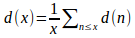## Averages of Arithmetical Functions

The values of arithmetical functions may function widely. For example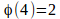but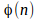can be arbitrarily large. It is often more useful to study a mean of the fuction. One such is defined as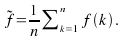Example

The mean of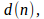where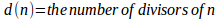is given by